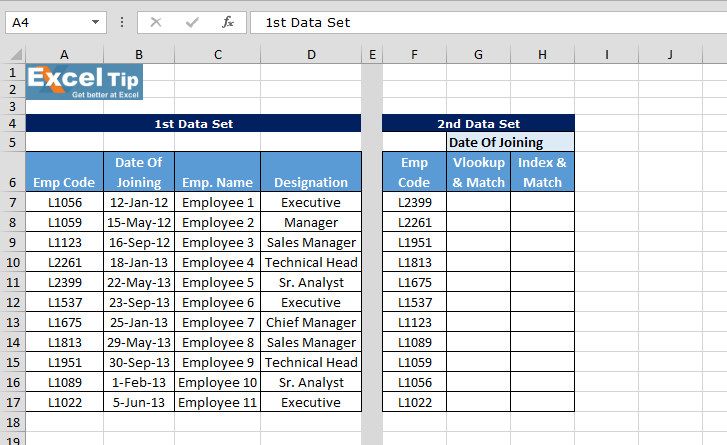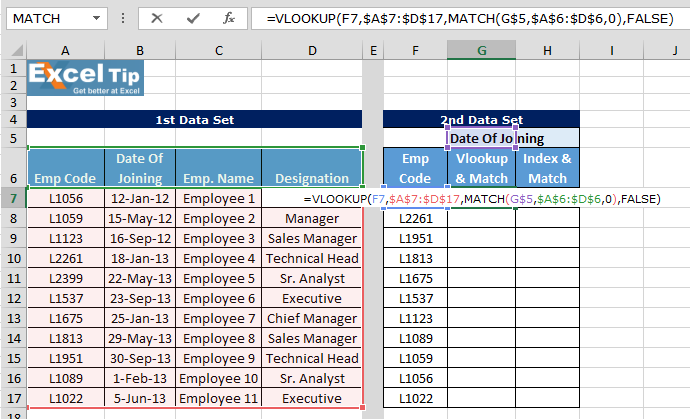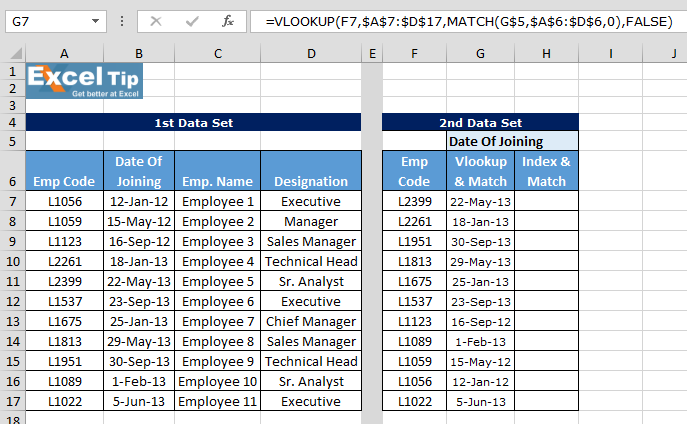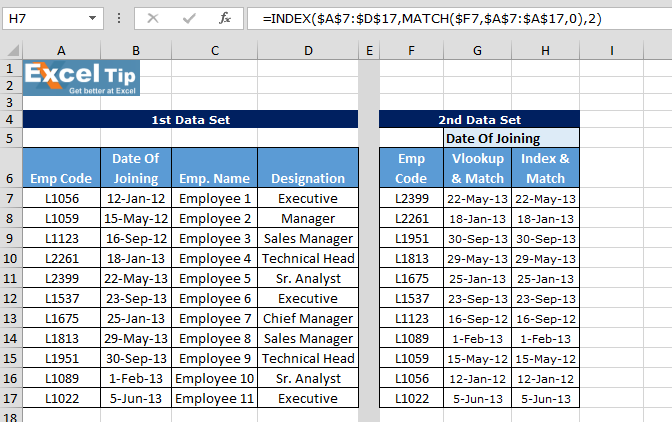# Index Match Formula VS Match Vlookup in Excel

In this article, we will learn to use Index Match formula at the place of Vlookup and Match formula in Microsoft Excel.

How the “INDEX” formula calculates and returns results?

Index function returns a value from the intersection between the row number and the column number in an Array. There are 2 syntaxes for the “INDEX” function.

What are the advantages of using the “INDEX” formula instead of “VLOOKUP” formula?

Let’s take an example to understand:

We have employee’s data in which we have few details: Employee code, Date of joining, Employee name, Designation.

In the 2nd data we want to pick joining date from the first data.How to pick the value by using Vlookup along with Match function?

Follow below given steps and formula:

• Enter the formula in cell G7
• =VLOOKUP(F7,\$A\$7:\$D\$17,MATCH(G\$5,\$A\$6:\$D\$6,0),FALSE)• Press Enter
• The function will return joining date
• Copy the same formula in the range G8:G17Formula Explanation:

• =VLOOKUP(F7,\$A\$7:\$D\$17, this part of formula will help to lookup the value
• MATCH(G\$5,\$A\$6:\$D\$6,0),FALSE), this part of formula will match the column criteria and then pick the value

How to pick the value by using Index and Match function?

Follow below given steps and formula:

• Enter the formula in cell H7
• =INDEX(\$A\$7:\$D\$17,MATCH(\$F7,\$A\$7:\$A\$17,0),2)• Press Enter
• The function will return same result as we got by using Vlookup & Match functionFormula Explanation:-

• =INDEX(\$A\$7:\$D\$17, in this part of function we have given the array range
• MATCH(\$F7,\$A\$7:\$A\$17,0),2), this part will match the common value accordingly will pick the value

The main difference between the two formulas is that while using Index there is no need to organize the data table into a special format – you simply find the intersection between a row and a column. While using Vlookup, you always need to move the lookup column to the left side of the data table.

1.•